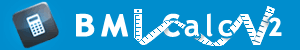BMR Calculator for Men

* Based on Harris Benedict equation

BMR calculator for men is an indispensable tool for determination of the daily calorie needs so as the weight were unaffected. However, men must know that only dietician can take into account all metabolic particularities of the body and carry out the final calculations. This BMR calculator for males can be used for men from 2 to 99 years of age. Our BMR calculator for men works on the basis of Harris-Benedict equation. In 1984, Harris Benedict Equation was reevaluated by Roza and Shizgal. Therefore, men can choose between two approaches to BMR calculations. This unique calculator for men determines the BMR. Moreover, the tool can also calculate the daily calorie needs depending on the activity and way of life.

Share

Imperial BMR Calculator for Men

Select Equation Source

Input or Select Age

years

or

Input or Select Height

feet

inch(es)

or

Input or Select Weight

pounds

or 1 stone = 14 pounds

Select Activity Level

Result

Metric BMR Calculator for Men

Select Equation Source

Input or Select Age

years

or

Input or Select Height

meter

centimeters

or

Input or Select Weight

kilograms

or

Select Activity Level

Result